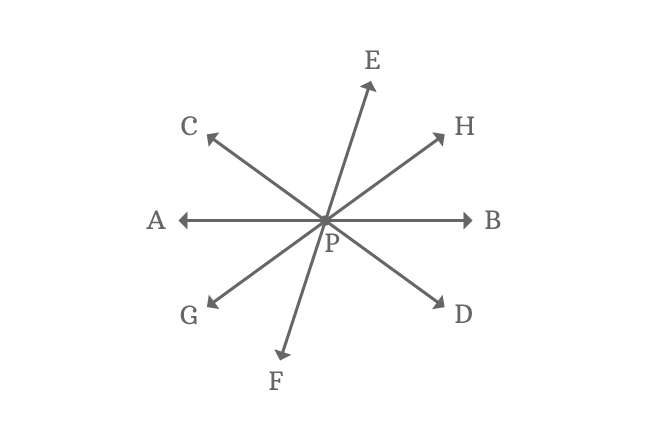# Concurrent lines

Three or more straight lines which intersect at a point in a plane are called concurrent lines.

## Introduction

Straight lines often pass through a point and it is very common in geometry. In some cases, three or more straight lines often pass through a point in a plane, and these straight lines are called concurrent lines.

### Example$\overleftrightarrow{AB}$, $\overleftrightarrow{CD}$, $\overleftrightarrow{EF}$ and $\overleftrightarrow{GH}$ are four lines they are passed through a point $P$ in a plane.

The total number of intersecting lines are four and it is more than two. So, the intersecting lines are called concurrent lines in geometry.

A best free mathematics education website that helps students, teachers and researchers.

###### Maths Topics

Learn each topic of the mathematics easily with understandable proofs and visual animation graphics.

###### Maths Problems

A math help place with list of solved problems with answers and worksheets on every concept for your practice.

Learn solutions

###### Subscribe us

You can get the latest updates from us by following to our official page of Math Doubts in one of your favourite social media sites.